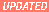23 feb 99, P. Cvitanovic'

# Chaos, Complexity and Computation

### U. Chicago Physics_251 Class Notes - version of Dec 16, 1998

This is computational dynamical systems course - might be useful if you chose to do your computations using Java.
• Front Page (3k)
• Contents (5k)
• (1) Intro Chapter (88k)
You might want to check this one out if you want to learn how to program Java applets to bounce billiard balls. Java can be picked up on the sun website. For examples of applets, check Gamelan.
• (2) Maps to Chaos (58k)
Program a Java applet to iterate logistic map.
• (3) Drawing Graphs (69k)
Find periodic orbits of the logistic map by the Newton-Raphson method; determine the stability of an orbit.
• (4) Fractals 1 (55k)
See the fractal nature of some natural processes, including the period-doubling sequence of the logistic map. Box counting dimension.
• (5) Fractals 2 (250k) Period-doubling bifurcation sequence of one-dimensional maps is universal; calculate the scaling exponents.
• (6) Orbits (67k) Numerical methods to compute the motion of particles governed by Newton's laws.
• (7) Differential Equations (142k)
• (8) Hamiltonian System (48k)